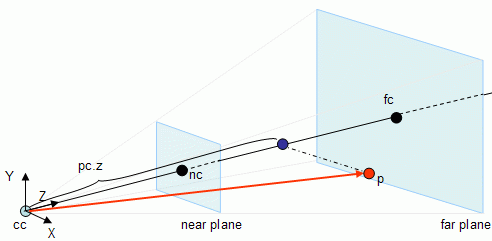Prev: Implementation Details Next: Testing Points II

The approaches presented in the previous sections were based on the fact that the volume of the view frustum is delimited by six planes. In those approaches the setup phase was responsible for extracting the planes equations, and testing was performed against the six planes. In this section a different approach is taken. This method is based on an article in Game Programming Gems 5 where the origin of the name is explained.

Consider that the camera has a referential based on the three unit vectors: X, Y and Z as shown in the next figure. Notice that the referential in the figure is not a right hand system (as in OpenGL), because the orientation of Z has been reversed to make the tutorial more intuitive.

For a given point p to be tested against the view frustum, the goal is to find its coordinates in this referential and then use this information to find out if the point is inside or outside the frustum.First the Z coordinate is checked. If the Z coordinate is not between the values of nearDist and farDist then the point is certainly outside of the view frustum, otherwise coordinates X and Y must be tested.In the image above, p is the point being tested. Consider pc as being the point p in camera referential coordinates. In order to find the Z coordinate of pc, pc.z first it is necessary to find the vector that goes from cc (the camera center) to p, and then the length of the projection of this vector on Z (the projection is the blue point) must be computed. As shown in the lines section of the maths tutorial, this can be done with a dot product (this is valid because it is assumed that Z is a unit vector), hence:

```	v = p - cc
pc.z = v . Z```

If pc.z is not between nearDist and farDist then p is outside the frustum.

```	if (pc.z > farDist || pc.z < nearDist)
return (OUTSIDE);```

Up to this point the value of pc.z is known, where pc is the value of point p in the camera referential coordinates. To find pc.y and pc.x a similar procedure is used: find the length of the projection of vector v in both the Y and X axis respectively.

```	v = p - cc
pc.z = v . Z
pc.y = v . Y
pc.x = v . X```

 Prev: Implementation Details Next: Testing Points II

### One Response to “Radar Approach – Testing Points”

1.It should probably be noted that in practice, if you test vertices of your model against the frustum using the Radar method, the vertex has to be multiplied with the model matrix.

` p = mModel * vertex `

Model, not Model-View!

This site uses Akismet to reduce spam. Learn how your comment data is processed.